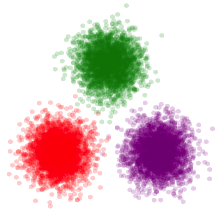# JuliaStats

Statistics and Machine Learning made easy in Julia.

• Easy to use tools for statistics and machine learning.
• Extensible and reusable models and algorithms
• Efficient and scalable implementation
• Community driven, and open source

## Packages

We bring together a number of great packages
Use the StatsKit meta-package to load all essential packages for statistics

### StatsBase

Basic functionalities for statistics

• Descriptive statistics and moments
• Sampling with/without replacement
• Counting and ranking
• Autocorrelation and cross-correlation
• Weighted statistics

### StatsModels

Interfaces for statistical models

• Formula and model frames
• Essential functions for statistical models

### DataFrames

Essential tools for tabular data

• DataFrames to represent tabular datasets
• Database-style joins and indexing
• Split-apply-combine operations, pivoting

### Distributions

Probability distributions

• A large collection of univariate, multivariate distributions
• descriptive stats, pdf/pmf, and mgf
• Efficient sampling
• Maximum likelihood estimation

### MultivariateStats

Multivariate statistical analysis

• Linear regression (LSQ and Ridge)
• Dimensionality reduction (PCA,CCA,ICA,...)
• Multidimensional scaling
• Linear discriminant analysis

### HypothesisTests

Hypothesis tests

• Parametric tests: t-tests
• Nonparametric tests: binomial tests, sign tests, exact tests, U tests, rank tests, etc

### MLBase

Swiss knife for machine learning

• Data preprocessing
• Score-based classification
• Performance evaluation
• Model selection, cross validation

### Distances

Various distances between vectors

• A large variety of metrics
• Efficient column-wise and pairwise computation
• Support weighted distances

### KernelDensity

Kernel density estimation

• Kernel density estimation for univariate and bivariate data
• User customization of interpolation points, kernel, and bandwidth

### Clustering

Algorithms for data clustering

• K-means
• K-medoids
• Affinity propagation
• Evaluation of clustering performance

### GLM

Generalized linear models

• Friendly API for fitting GLM to data
• Work with data frames and formulas
• A variety of link types
• Optimized implementation

### NMF

Nonnegative matrix factorization

• A variety of NMF algorithms, including Lee & Seung's, Projected ALS and projected gradient, with optimized implementation.
• NNDSVD initialization

### RegERMs

Lasso/Elastic Net linear and generalized linear models

• glmnet coordinate descent algorithm
• Polynomial trend filtering
• O(n) fused Lasso
• Gamma Lasso (a concave regularization path glmnet variant)

### TimeSeries

Time series analysis

• Tools to represent, manipulate, and apply computation to time series data

## Community

We have an active and friendly community.

Github page: https://github.com/JuliaStats